# Simulating Rubik Cube Actions with Java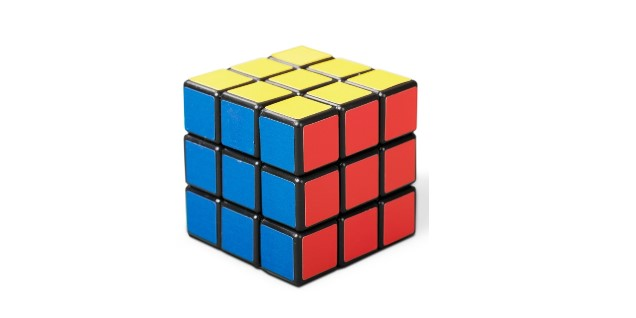Let’s work on something fun today, simulating a rubik’s cube. This is the precursor to my next topic to provide the solution to solve rubik’s cube.

## Modelling

There can be many ways we can simulate the rubik’s cube model as a class, but my goal here is to make it as simple and easy to understand as possible. A seemingly more scientific way is to have 3 dimensional data, but that will involve some 3D maths which can get confusing, so I’m not going to do that. Instead, I will break down the rubik’s cube into 6 sides, and lay it flat.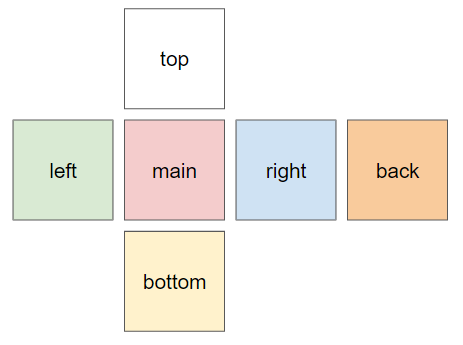So we declare a class named `RubikSide` to model it, with the size of the cube and the value for each position to represent the color. We are just doing a 3x3 rubik’s cube, but the code should be able to handle different sizes. Instead of saving the 6 colors, we just use numbers 1 - 6 to represent the colors - red, blue, orange, green, white, and yellow.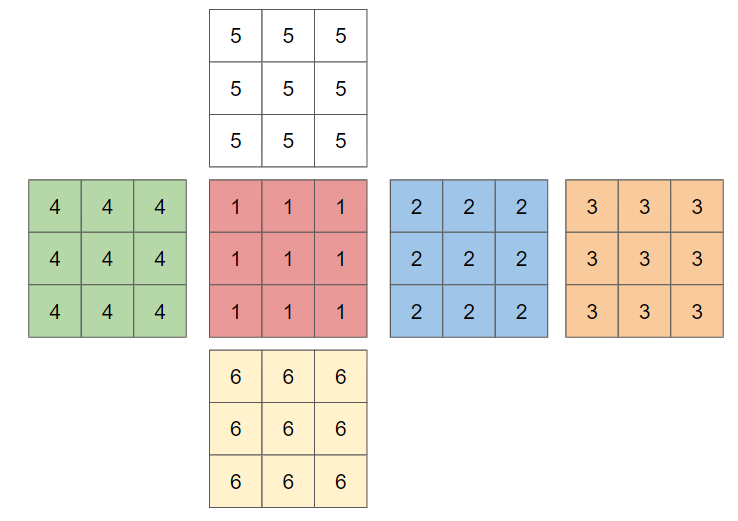``````public class RubikSide implements Cloneable{
private final int size;
private int[][] values;

public RubikSide(int size, int value){
this.size = size;
int[] dimension = IntStream.generate(() -> value).limit(size).toArray();
values = IntStream.range(0, size)
.boxed()
.map(i -> dimension.clone())
.toArray(int[][]::new);
}
}
``````

The values for each side are stored in the 2D array named `values`. The 1st dimension is the row, and the 2nd dimension is the column.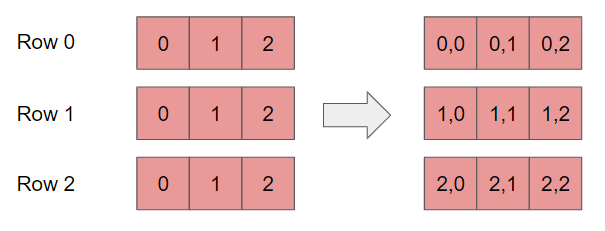As we combine all 6 sides to form the rubik’s cube, we define that the position should be as such when laid out flat. This is very important to our later works, especially when we turn the columns up and down.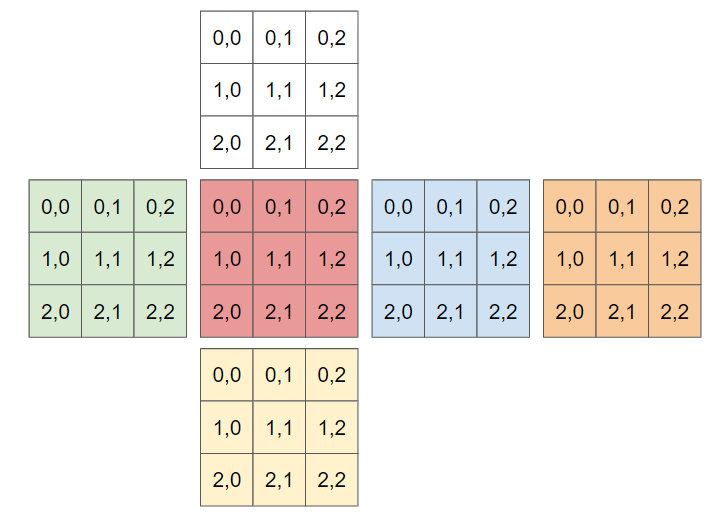``````public class RubikCube{
private RubikSide main;
private RubikSide right;
private RubikSide left;
private RubikSide back;
private RubikSide top;
private RubikSide bottom;
private int size;

public RubikCube(int size){
this.size = size;
main = new RubikSide(size, 1);
right = new RubikSide(size, 2);
back = new RubikSide(size, 3);
left = new RubikSide(size, 4);
top = new RubikSide(size, 5);
bottom = new RubikSide(size, 6);
}

}
``````

## Basic Functions

To prepare for the actions we can perform on the rubik’s cube, we should create some methods to get and set the rows and columns of each `RubikSide`. Getting the rows is pretty straightforward, and getting the column just need a little manipulation.

``````public int[] getRow(int row){
return values[row];
}

public int[] getCol(int col){
return IntStream.range(0, size)
.map(i -> values[i][col])
.toArray();
}
``````

Setting the values is similarly almost the same.

``````public void setRow(int row, int[] newValues){
values[row] = newValues;
}

public void setCol(int col, int[] newValues){
IntStream.range(0, size).forEach(i -> values[i][col] = newValues[i]);
}
``````

## Actions

Now we are ready to create the actions we can perform on the rubik’s cube. My strategy for simplification is to only target the actions that can be perform on the `main` side. Without specifying the rows and columns, which should be dynamic, as we want to cater to different rubik’s cube sizes (3x3, 4x4, 5x5), I can summarize all the actions available for 1 side to be as follows:

1. Turn row `X` left
2. Turn row `X` right
3. Turn column ‘Y’ up
4. Turn column ‘Y’ down
5. Rotate `main` side clockwise
6. Rotate `main` side anti-clockwise.

### Turning Row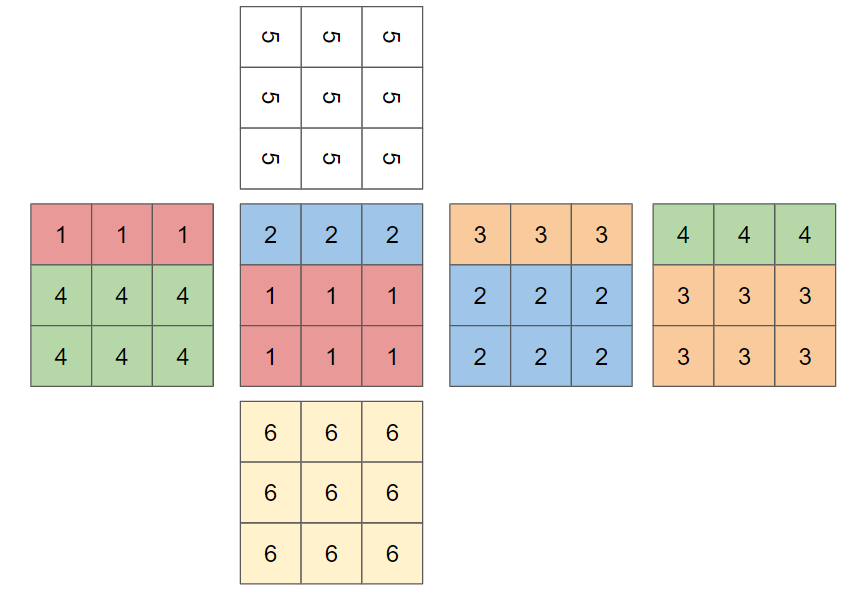Turning row left and right is the easiest, I just need to reassign the values of each side. For example, turning a row to the left is just reassigning the values of the row on the `main` side with the values of the same row on the `right` side. Then we replace the values of the row on the `right` side with the values of the same row on the `back` side. And we replace the values of the row on the `back` side with the values of the row on the `left` side. Lastly, before we begin all these, we should have a copy of the values of the row on the `main` side, so that we can replace the values of the row on the `left` side with the values of the row on the `main` side. However, we need to make sure that if the top or bottom row is involved, we need to rotate the respective top and bottom sides.

``````public void turnRowToRight(int row) throws Exception{
int[] mainTopRow = getMain().getRow(row);
getMain().setRow(row, getLeft().getRow(row));
getLeft().setRow(row, getBack().getRow(row));
getBack().setRow(row, getRight().getRow(row));
getRight().setRow(row, mainTopRow);
if(row == 0){
getTop().rotateAntiClockwise();
}else if(row == (getSize() - 1)){
getBottom().rotateClockwise();
}
}

public void turnRowToLeft(int row) throws Exception{
int[] mainTopRow = getMain().getRow(row);
getMain().setRow(row, getRight().getRow(row));
getRight().setRow(row, getBack().getRow(row));
getBack().setRow(row, getLeft().getRow(row));
getLeft().setRow(row, mainTopRow);
if(row == 0){
getTop().rotateClockwise();
}else if(row == (getSize() - 1)){
getBottom().rotateAntiClockwise();
}
}
``````

So now, we need to create the rotation function in the `RubikSide`. Rotating clockwise can be visualised as such.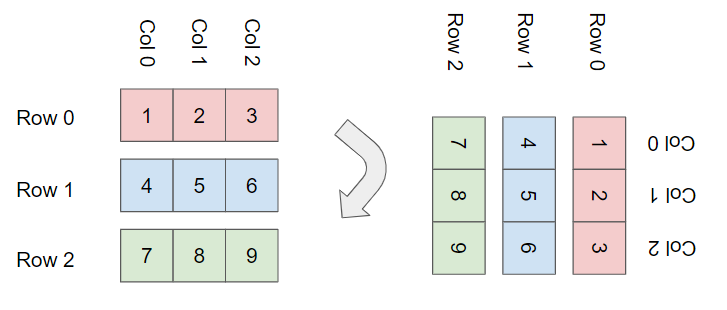As can be seen from the above diagram, the new rows are just the reversal of each column, so we can create the `rotateClockwise` function easily as such.

``````public void rotateClockwise(){
values = IntStream.range(0, size)
.boxed()
.map(i -> Utils.reverseArray(getCol(i)))
.toArray(int[][]::new);
}
``````

Noticed that we refactor out the `reverseArray` function to a utility class to ensure compliance to the SOLID principle.

``````public class Utils {
public static int[] reverseArray(int[] arr){
return IntStream.rangeClosed(1, arr.length)
.map(i -> arr[arr.length - i])
.toArray();
}
}
``````

To rotate anti-clockwise, our new `row 0` is our `column n`, and our `row n` is our `column 0`, as can be visualised as such.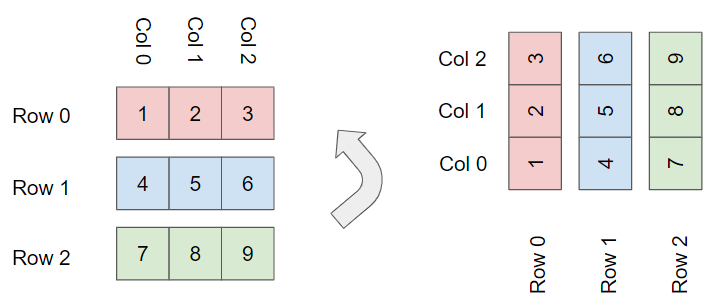``````public void rotateAntiClockwise(){
values = IntStream.rangeClosed(1, size)
.boxed()
.map(i -> getCol(size - i))
.toArray(int[][]::new);
}
``````

### Turning Column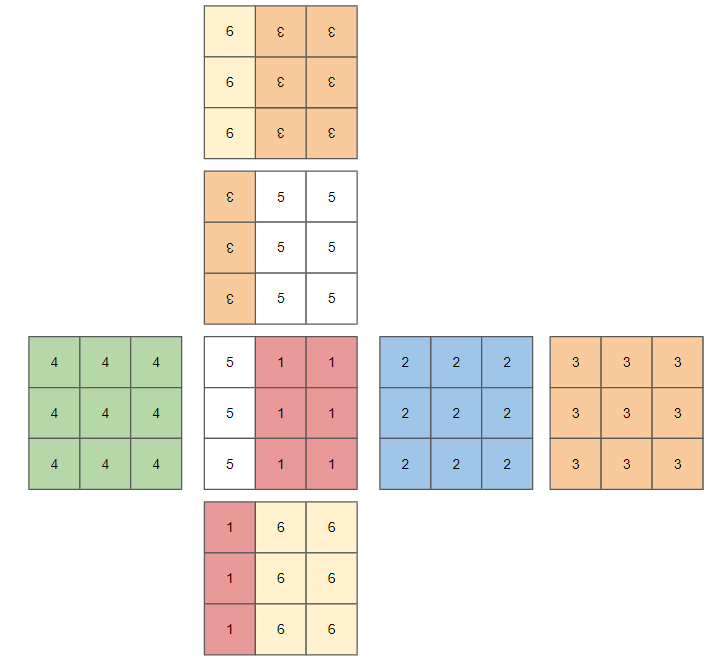We want to apply the same logic of turning rows to turning columns. So similarly, to turn a column down, we first save a copy of the values of the column on the `main` side. Then we replace the values of the column on the `main` side with the values of the column on the `top` side, and replace the values of the column on the `top` side with the values of the column on the `back` side. Continuing on, we replace the values of the columns on the `back` side with the values of the `column` on the `bottom` side, and replace the values of the column on the `bottom` side with the values of the column on the `main` side which we have saved earlier. And if it is the first or last column, we need to rotate the values on the `left` and `right` side.

However, there is a litte tricky portion if we want to use this method, because the top part of the `back` face is now the bottom, and the left part of the `back` is now the right.We need to flip the `back` face, before we can do a simple reassignment of values, so we supplied a `reverse2dArray` funtion.

``````public static int[][] reverse2dArray(int[][] arr){
int[][] interim =  IntStream.range(0, arr.length)
.boxed()
.map(i -> reverseArray(arr[i]))
.toArray(int[][]::new);

return IntStream.rangeClosed(1, interim.length)
.boxed()
.map(i -> interim[interim.length - i])
.toArray(int[][]::new);
}
``````

Then we can use this new function to temporary alter the values of the `back` face into the perspective we need so that we can do a simple column reassignment. But we need to switch the perspective back as soon as we have finished the column assignment.

``````public void turnColUp(int col) throws Exception{
int[] mainCol = getMain().getCol(col);
RubikSide reversedBack = getBack().cloneReversed();
getMain().setCol(col, getBottom().getCol(col));
getBottom().setCol(col, reversedBack.getCol(col));
reversedBack.setCol(col, getTop().getCol(col));
getTop().setCol(col, mainCol);
back = reversedBack.cloneReversed();
if(col == 0){
getLeft().rotateAntiClockwise();
}else if(col == (getSize() - 1)){
getRight().rotateClockwise();
}
}

public void turnColDown(int col) throws Exception{
int[] mainCol = getMain().getCol(col);
RubikSide reversedBack = getBack().cloneReversed();
getMain().setCol(col, getTop().getCol(col));
getTop().setCol(col, reversedBack.getCol(col));
reversedBack.setCol(col, getBottom().getCol(col));
getBottom().setCol(col, mainCol);
back = reversedBack.cloneReversed();
if(col == 0){
getLeft().rotateClockwise();
}else if(col == (getSize() - 1)){
getRight().rotateAntiClockwise();
}
}
``````

## Rotating `main` side

As our goal is to keep things simple and clear, we want to focus all operations just on the `main` side. For the rotating of the `main` side, it is the same as turning the 1st column of the `right` side. But we don’t want to do operations on the other sides, yet we still need to provide all operations possible. Therefore, my solution is to not have a rotate action, but `face` action to turn other sides to be the `main` side.

## Face Action

This should be the easiest action by just replacing the sides. And we only need to provide 5 of such `face` actions - `face right`, `face back`, `face left`, `face top`, and `face bottom`.

``````public void face(FACE newFace){
Map<FACE, RubikSide> old = Map.of(
FACE.MAIN, getMain().clone(),
FACE.RIGHT, getRight().clone(),
FACE.BACK, getBack().clone(),
FACE.LEFT, getLeft().clone(),
FACE.TOP, getTop().clone(),
FACE.BOTTOM, getBottom().clone()
);
main = getFace(newFace);
right = old.get(getRightFaceOf(newFace));
back = old.get(getBackFaceOf(newFace));
left = old.get(getLeftFaceOf(newFace));
top = old.get(getTopFaceOf(newFace));
bottom = old.get(getBottomFaceOf(newFace));
}

public static FACE getBackFaceOf(FACE face){
if(face == FACE.MAIN) return FACE.BACK;
if(face == FACE.RIGHT) return FACE.LEFT;
if(face == FACE.BACK) return FACE.MAIN;
if(face == FACE.LEFT) return FACE.RIGHT;
if(face == FACE.TOP) return FACE.BOTTOM;
return FACE.TOP;
}

public static FACE getRightFaceOf(FACE face){
if(face == FACE.MAIN) return FACE.RIGHT;
if(face == FACE.RIGHT) return FACE.BACK;
if(face == FACE.BACK) return FACE.LEFT;
if(face == FACE.LEFT) return FACE.MAIN;
return FACE.RIGHT;
}

public static FACE getLeftFaceOf(FACE face){
if(face == FACE.MAIN) return FACE.LEFT;
if(face == FACE.RIGHT) return FACE.MAIN;
if(face == FACE.BACK) return FACE.RIGHT;
if(face == FACE.LEFT) return FACE.BACK;
return FACE.LEFT;
}

public static FACE getTopFaceOf(FACE face){
if(face == FACE.TOP) return FACE.BACK;
if(face == FACE.BOTTOM) return FACE.MAIN;
return FACE.TOP;
}

public static FACE getBottomFaceOf(FACE face){
if(face == FACE.TOP) return FACE.MAIN;
if(face == FACE.BOTTOM) return FACE.BACK;
return FACE.BOTTOM;
}
``````

### Defining and Performing Actions

Since the motivation of modelling the rubik’s cube is to solve it, we want a way to easily call any of the actions. A simple way is to just put all possible actions in a list, so that each action is assigned a number. But as our rubik’s cube size is dynamic, the number of possible actions is also dynamic, depending on the number of rows and columns.

Next, we have 2 very different types of actions - `Turn` and `Face`. But they are both actions, so we generalize them as an interface - `RubikCubeAction`.

``````public interface RubikCubeAction {
String getName();
void performAction(RubikCube rubikCube);
RubikCubeAction oppositeAction();
}
``````

We provide 3 functions here: `getName`, `performAction`, and `oppositeAction`. The `getName` is supposed to print the name of the action so that we can visualize what happen. The `performAction` utilises a strategy pattern to call the different actions with the same method without a long list of if-else or switch statements. Lastly, in order to make sure our actions are updating the values correctly, we want to run a whole list of actions, then run the reverse list of each action’s opposite action, and it should give us back the perfect rubik’s cube. So we need each action to have an opposite action.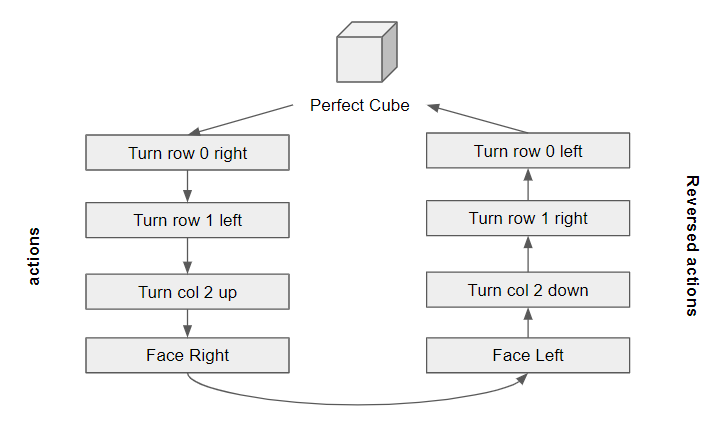And so we have a `FaceAction` and a `TurnAction` that implements the `RubikCubeAction`. And each of these 2 actions can help to provide all the possible actions for the cube.

``````public class FaceAction implements RubikCubeAction{

private final RubikCube.FACE face;

private FaceAction(RubikCube.FACE face){
this.face = face;
}

public static FaceAction[] allActions(){
return Arrays.stream(RubikCube.FACE.values())
.filter(face -> face != RubikCube.FACE.MAIN)
.map(FaceAction::new)
.toArray(FaceAction[]::new);
}

@Override
public void performAction(RubikCube rubikCube){
rubikCube.face(face);
}

...
}

``````
``````public class TurnAction implements RubikCubeAction{

public enum DIRECTION { LEFT, RIGHT, UP, DOWN}
public enum TURN_TYPE { ROW, COL }
public int turnPosition;
public DIRECTION direction;
public TURN_TYPE turnType;

private TurnAction(TURN_TYPE turnType, DIRECTION direction, int turnPosition){
this.turnType = turnType;
this.direction = direction;
this.turnPosition = turnPosition;
}

public static TurnAction[] allActions(int size){
return IntStream.range(0, size)
.boxed()
.flatMap(i -> Stream.of(
new TurnAction(TURN_TYPE.ROW, DIRECTION.LEFT, i),
new TurnAction(TURN_TYPE.ROW, DIRECTION.RIGHT, i),
new TurnAction(TURN_TYPE.COL, DIRECTION.UP, i),
new TurnAction(TURN_TYPE.COL, DIRECTION.DOWN, i)
))
.toArray(TurnAction[]::new);
}

@Override
public void performAction(RubikCube rubikCube){
try {
if(turnType == TURN_TYPE.COL){
if(direction == DIRECTION.UP) rubikCube.turnColUp(turnPosition);
if(direction == DIRECTION.DOWN) rubikCube.turnColDown(turnPosition);
}else if(turnType == TURN_TYPE.ROW){
if(direction == DIRECTION.LEFT) rubikCube.turnRowToLeft(turnPosition);
if(direction == DIRECTION.RIGHT) rubikCube.turnRowToRight(turnPosition);
}
}catch (Exception e){
e.printStackTrace();
}
}
...
}
``````

As of the strategy pattern, we just need a simple call from the `RubikCubeAction` interface to execute the action in the `RubikCube`.

``````public void performAction(RubikCubeAction action) {
action.performAction(this);
}
``````

Lastly we can define all the possible actions in the constructor of the `RubikCube`.

``````private RubikCubeAction[] allActions;

public RubikCube(int size){
this.size = size;
main = new RubikSide(size, 1);
right = new RubikSide(size, 2);
back = new RubikSide(size, 3);
left = new RubikSide(size, 4);
top = new RubikSide(size, 5);
bottom = new RubikSide(size, 6);
allActions = Stream.concat(
Stream.of(FaceAction.allActions()),
Stream.of(TurnAction.allActions(size)))
.toArray(RubikCubeAction[]::new);
}
}
``````

## Verifying

### Testing the Reverse Action List Hypothesis

To ensure what we did so far are correct, we create a `RubikSolution` to initialize a 3x3 rubiks cube, and perform a list of random actions, and print out how the values of each side of the cube. Then we run a reverse list of the actions perform and print the cube again. We should get a perfect cube.

### Print

In order to make it easier to visualize the outcome of the actions, let’s provide a print method.

``````public void print(){
String[] box = join(RubikSide.getEmptyString(getSize()), getTop().getString());
Arrays.stream(box).forEach(System.out::println);

box = join(getLeft().getString(), getMain().getString(), getRight().getString(), getBack().getString());
Arrays.stream(box).forEach(System.out::println);

box = join(RubikSide.getEmptyString(getSize()), getBottom().getString());
Arrays.stream(box).forEach(System.out::println);

System.out.println(" ");
}
``````

### Random Actions

``````public List<RubikCubeAction> randomActions(RubikCube rubikCube, int count){
Random random = new Random();
int actionCount = rubikCube.getAllActions().length;
return IntStream.range(0, count).boxed().map(i -> {
RubikCubeAction action = rubikCube.getAllActions()[random.nextInt(actionCount)];
rubikCube.performAction(action);
return action;
}).toList();
}
``````

### Reverse actions

``````public List<RubikCubeAction> reverseActions(List<RubikCubeAction> originalActions){
return IntStream.rangeClosed(1, originalActions.size())
.boxed()
.map(i -> originalActions.get(originalActions.size() - i))
.map(RubikCubeAction::oppositeAction)
.toList();
}
``````

### Running the Hypothesis

``````public static void main(String[] args) {
RubikCube cube = new RubikCube(3);
RubikSolution solution = new RubikSolution();
List<RubikCubeAction> randomActions = solution.randomActions(cube, 20);
randomActions.forEach(action -> System.out.println(action.getName()));
cube.print();
System.out.println(cube.check());

List<RubikCubeAction> reverseActions = solution.reverseActions(randomActions);
reverseActions.forEach(action -> System.out.println(action.getName()));
reverseActions.forEach(cube::performAction);
cube.print();
System.out.println(cube.check());
}
``````

We got a list of actions

``````TURN_ROW_0_RIGHT
TURN_ROW_1_LEFT
FACE_RIGHT
TURN_COL_2_DOWN
FACE_TOP
FACE_LEFT
TURN_COL_1_UP
TURN_ROW_0_LEFT
TURN_COL_2_UP
FACE_LEFT
FACE_TOP
TURN_ROW_1_LEFT
TURN_COL_1_DOWN
TURN_COL_2_DOWN
TURN_ROW_0_LEFT
FACE_BACK
TURN_COL_2_DOWN
TURN_ROW_0_RIGHT
TURN_COL_2_DOWN
FACE_RIGHT
``````

And print out the rubik’s cube.

``````          [4, 2, 4]
[5, 1, 5]
[3, 4, 2]
[2, 6, 5] [5, 3, 6] [6, 2, 3] [1, 2, 5]
[3, 6, 4] [6, 2, 1] [3, 5, 4] [5, 4, 2]
[4, 1, 1] [3, 5, 6] [1, 1, 3] [5, 3, 1]
[4, 6, 2]
[6, 3, 4]
[6, 1, 2]

``````

Then we got the reversed list of opposite actions

``````FACE_LEFT
TURN_COL_2_UP
TURN_ROW_0_LEFT
TURN_COL_2_UP
FACE_BACK
TURN_ROW_0_RIGHT
TURN_COL_2_UP
TURN_COL_1_UP
TURN_ROW_1_RIGHT
FACE_BOTTOM
FACE_RIGHT
TURN_COL_2_DOWN
TURN_ROW_0_RIGHT
TURN_COL_1_DOWN
FACE_RIGHT
FACE_BOTTOM
TURN_COL_2_UP
FACE_LEFT
TURN_ROW_1_RIGHT
TURN_ROW_0_LEFT
``````

And we got back our perfect rubik’s cube!

``````          [5, 5, 5]
[5, 5, 5]
[5, 5, 5]
[4, 4, 4] [1, 1, 1] [2, 2, 2] [3, 3, 3]
[4, 4, 4] [1, 1, 1] [2, 2, 2] [3, 3, 3]
[4, 4, 4] [1, 1, 1] [2, 2, 2] [3, 3, 3]
[6, 6, 6]
[6, 6, 6]
[6, 6, 6]

``````

The code for this topic is available on https://github.com/thecodinganalyst/RubiksCube.

Tags:

Categories:

Updated: